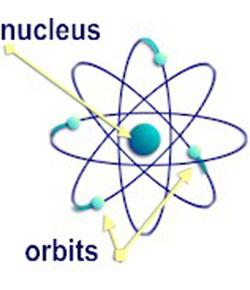# Unit IV: Test 2: Nuclear Physics

3 Questions | Total Attempts: 845SettingsNuclear Physics Topic Test

Related Topics
• 1.
The ionization power of the three radiations from radioactive substances arranged in ascending order is
• A.

Alpha, beta, gamma

• B.

Alpha, gamma, beta

• C.

Beta, alpha, gamma

• D.

Gamma, beta alpha

• 2.
The reading of a detector of radioactive radiation falls from an average rate of 800 per minute to an average of 1000 per minute in a period of 24 minutes. The half-life is
• A.

3 minutes

• B.

6 minutes

• C.

8 minutes

• D.

12 minutes

• 3.
An energy of 7.20E8 J has a rest mass equivalence of
• A.

8.00E-9 kg

• B.

2.40E0 kg

• C.

1.25E8 kg

• D.

6.48E25 kg